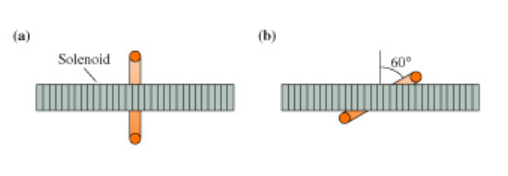# Problem: The 2.0-cm-diameter solenoid in the figure passes through the center of a 6.0-cm-diameter loop. The magnetic field inside the solenoid is 0.20What is the magnetic flux through the loop when it is perpendicular to the solenoid?Express your answer using two significant figures.Magnetic Flux = ______ Wb

###### FREE Expert Solution

Magnetic flux through a surface:

r = D/2 = (2.0 cm/2)(1m/100cm) = 0.01 m

80% (73 ratings)###### Problem Details

The 2.0-cm-diameter solenoid in the figure passes through the center of a 6.0-cm-diameter loop. The magnetic field inside the solenoid is 0.20

What is the magnetic flux through the loop when it is perpendicular to the solenoid?

Magnetic Flux = ______ Wb